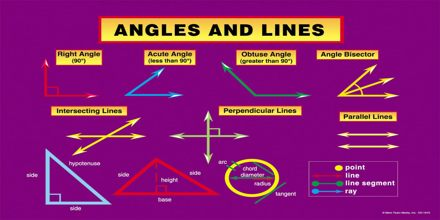Mathematic

# Geometry VocabularyBasic purpose of this lecture is to present on Geometry Vocabulary. Geometry is the study of shapes. They studied Geometry in Ancient Mesopotamia and Ancient Egypt. Geometry is important in the art and construction fields. Here describe some common Geometry Vocabulary. Like; Square: A quadrilateral with 4 equal sides and 4 right angles. Rectangle: A Quadrilateral with 4 sides and 4 right angles. Triangle: A polygon with 3 sides and 3 angles. Circle: A polygon made with a continuous line which is always the same distance from the center.